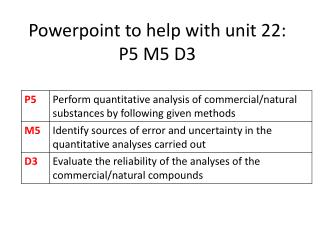DownloadDownload PresentationPowerpoint to help with unit 22: P5 M5 D3

# Powerpoint to help with unit 22: P5 M5 D3

Download Presentation## Powerpoint to help with unit 22: P5 M5 D3

- - - - - - - - - - - - - - - - - - - - - - - - - - - E N D - - - - - - - - - - - - - - - - - - - - - - - - - - -
##### Presentation Transcript

1. Powerpoint to help with unit 22: P5 M5 D3

2. P5: Perform quantitative analysis of commercial / natural substances by following given methods • Two practicals (along with their calculations) to carry out. These two practicals are: • Extracting salt from butter and then using titration to analyse the salt extracted • Extracting limonene (an oil) from oranges and then using titration to analyse the limonene extracted

3. When extracting salt from butter: • Sample results:

4. When analysing the extracting salt by titration • Sample results

5. Example of titration of salt butter calculations

6. When extracting limonene from oranges • Sample results

7. When analysing the extracted limonene by titration • Sample results

8. M5: Identify sources of error and uncertainty in the quantitative analyses carried out • When identifying sources of errors consider the following: • Were you able to completely melt or dissolve the butter? • Did you use the correct part of the orange AND cut the pieces small enough? • Were you able to separate out ALL of the desired / extracted chemicals? (e.g. issues with Separating funnel?) • Were you able to fully evaporate the desired chemicals? • Was it easy to detect the endpoints of titrations? • Did you over boil substances which could lead to burning some of the substances? • Did you fully dry the extracted substances?

9. Calculating percentage errors • Formula to use: % error = Maximum error X 100 Measurement

10. Apparatus that you used for P5 • 25ml glass pipette • Has a maximum error of 0.06ml • The measurement you made for the titrations was a volume of 25ml • Mass balance • Has a maximum error of 0.001g • You made several measurements with the mass balance. E.g: you measured the mass of an empty evaporating dish, the mass of butter used at the beginning

11. Burette • Has a maximum error of 0.05ml • You made measurements with the burette when you measured the initial burette reading and the final burette reading during the titrations • 250ml volumetric flask • Has a maximum error of 0.15g • The measurement you made when using the volumetric flask was a volume of 250ml

12. Example of how to calculate percentage error • Apparatus: 250ml volumetric flask • Maximum error of 250ml volumetric flask: 0.15ml • Measurement made: 250ml % error = Maximum error X 100 Measurement = 0.15 X 100 = 0.06% 250

13. Accuracy: The closeness of readings to the true value. Accuracy refers to the correctness of a single measurement. Accuracy is determined by comparing the measurement against the true or accepted value

14. Precision: The degree of uncertainty of a measurement; usually the size of the unit of measurement used. Precision refers to how well experimental data and values agree with each other in multiple tests

15. Reliability When an experiment is repeated with a different group of people or a different batch of the same chemicals and very similar results are obtained then those results are said to be reliable. Reliability is measured by a percentage – if you get exactly the same results every time then they are 100% reliable Results should be both accurate and precise in order to be reliable

16. How to decide if your results are reliable or unreliable Compare your results to others • Copy and paste results for a specific part of the practical (e.g. titration of limonene) from two other students . • Decide if your results and the other two students’ results are consistent (which could mean your results are reliable) or inconsistent (which could mean your results are unreliable). • Explain reasons for inconsistencies- (e.g. different masses of extracted substances used).

17. Consider the significant figures you used • Did you use significant figures in your calculations that were consistent with the significant figures that your measuring equipment reads to? Did you use consistent significant figures throughout your calculations? (If you did then this could mean your calculated results are reliable). • Did you use 3 significant figures- this is considered accurate for Science. Not using enough significant figures OR using too many significant figures could mean that errors are carried forward in calculations.

18. Consider what effect sources of errors would have on the reliability of the results • An example of an error: Not detecting the correct end point during the titration as you’ve gone over the end point (added too much chemical into the conical flask). • How might this error affect the reliability of results? It will make the results less accurate as the titre values will not be close to their true titre values BUT the results are likely to be precise if you are making the same mistake and going over the end point by the same amount.

19. For each error, decide what effect it would have on the numerical answer (would it make it larger or smaller?) • An example of an error: Not detecting the correct end point during the titration as you’ve gone over the end point (added too much chemical into the conical flask). • This error would cause the titre readings to be larger than what they should be